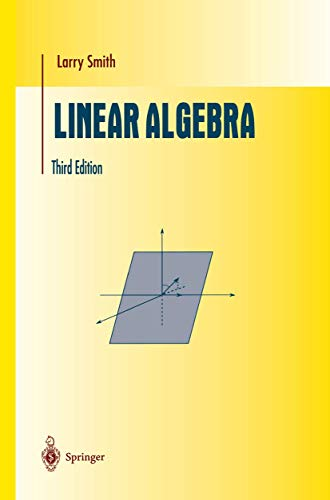• Free Shipping on all orders in Australia
• Over 7 million books in stock
• Proud to be B-Corp
• We aim to be carbon neutral by 2022
• Over 120,000 Trustpilot reviews
Item 1 of 0# Linear Algebra by Larry Smith

Condition - New
\$128.29
Only 2 left

## Summary

This popular and successful text was originally written for a one-semester course in linear algebra at the sophomore undergraduate level. A compact, but mathematically clean introduction to linear algebra with particular emphasis on topics in abstract algebra, the theory of differential equations, and group representation theory.

## Linear Algebra Summary

### Linear Algebra by Larry Smith

This popular and successful text was originally written for a one-semester course in linear algebra at the sophomore undergraduate level. Consequently, the book deals almost exclusively with real finite dimensional vector spaces, but in a setting and formulation that permits easy generalisation to abstract vector spaces. A wide selection of examples of vector spaces and linear transformation is presented to serve as a testing ground for the theory. In the second edition, a new chapter on Jordan normal form was added which reappears here in expanded form as the second goal of this new edition, after the principal axis theorem. To achieve these goals in one semester it is necessary to follow a straight path, but this is compensated by a wide selection of examples and exercises. In addition, the author includes an introduction to invariant theory to show that linear algebra alone is incapable of solving these canonical forms problems. A compact, but mathematically clean introduction to linear algebra with particular emphasis on topics in abstract algebra, the theory of differential equations, and group representation theory.

1: Vectors in the Plane and Space. 2: Vector Spaces. 3: Examples of Vector Spaces. 4: Subspaces. 5: Linear Independence and Dependence. 6: Finite Dimensional Vector Spaces and Bases. 7: The Elements of Vector Spaces: A Summing Up. 8: Linear Transformations. 9: Linear Transformations: Examples and Applications. 10: Linear Transformations and Matrices. 11: Representing Linear Transformations by Matrices. 12: More on Representing Linear Transformations by Matrices. 13: Systems of Linear Equations. 14: The Elements of Eigenvalue and Eigenvector Theory. 15: Inner Product Spaces. 16: The Spectral Theorem and Quadratic Forms. 17: Jordan Canonical Form. 18: Application to Differential Equations. 19: The Similarity Problem. Appendix A: Multilinear Algebra and Determinants. B: Complex Numbers.

NPB9781461272380
9781461272380
1461272386
Linear Algebra by Larry Smith
New
Paperback
Springer-Verlag New York Inc.
2012-10-17
454
N/A
Book picture is for illustrative purposes only, actual binding, cover or edition may vary.
This is a new book - be the first to read this copy. With untouched pages and a perfect binding, your brand new copy is ready to be opened for the first time